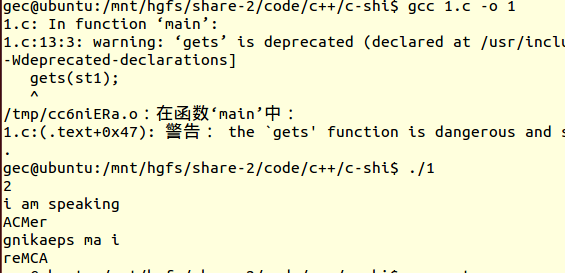Sine 的一个朋友疯了，最近他老爱说反话，别人说什么他就倒着说一遍，真是个奇怪的人。那他到底说了什么呢？

（他说的话不超过 1000 个字符。）

2

i am speaking

ACMer

gnikaeps ma i

reMCA

``````#include<stdio.h>
#include<string.h>
int main()
{
int i,m,n;
char st1;
char st2;
scanf("%d",&m);
while(m--)
{
gets(st1);
scanf("%s",st1);
i = strlen(st1);
for (n=0;i>= 0; n++)
{
st2[n] = st1[i];
i--;
}
st2[n] = '\0';
for(n=0;n<i;n++)
printf("%s",st2);
printf("\n");
}
return 0;
}

``````

3个回答

``````#include<stdio.h>
#include<string.h>
#define N 1000
int main()
{
int i,m,n;
char st1[N];
char st2[N];
memset(st1,0x0,N);
memset(st1,0x0,N);
scanf("%d",&m);
getchar();
while(m--)
{
gets(st1);
i = strlen(st1);
for (n=0;i>0;)
st2[n++] = st1[--i];
st2[n] = '\0';
printf("%s\n",st2);
}
return 0;
}

``````qtchen_1988 回复alwaysmissme: 肯定有用才打上去的，memset(st1,0x0,N);将字符串全部置‘\0'也就是初始化，getchar(); 是因为scanf输入时，输入缓冲区还有'\n'符，目的为了读掉'\n'.否则，第一次会没输入直接输出了
10 个月之前 回复10 个月之前 回复

2
i am speaking
ACMer

gnikaeps ma i
reMCA

123456
6位于数组第5个，但是这个数组长度是6，这里也需要注意。

``````#include<stdio.h>
#include<string.h>
int main()
{
int i,m,n;
char st1;
char st2;
scanf("%d",&m);
getchar();//去除按下的inter键，清理缓冲
n=0;
while(m--)
{
gets(st1);
i = strlen(st1)-1;//数组元素从0开始，但是长度从1开始
for(;i>=0;n++,i--)
{
st2[n] = st1[i];
}
st2[n] = '\0';
n++;
}
m=i=0;
while(m!=n)
{
m+=strlen(&st2[i])+1;
printf("%s\n",&st2[i]);
i=m;
}
return 0;
}

````````````#include "stdio.h"
#include "stdlib.h"

int main()
{
int i,m,n;
char st1;
char st2;
scanf("%d",&m);
while(m--)
{
gets(st1);
scanf("%s",st1);
i = strlen(st1);
i--;
for (n=0;i>=0; n++)
{
st2[n] = st1[i];
i--;
}
st2[n] = '\0';
//for(n=0;n<i;n++);
printf("%s\r\n",st2);
//printf("\n");
}
return 0;
}
``````10 个月之前 回复